Fluids Index

Fluid Flow in pipes

Pump Notes and Calculations Pumps
Valve Notes Valves
Fluid Power Notes Pneumatics ..... Hydrostatics

Introduction

The following notes should enable a mechanical engineer to establish basic flow conditions and head losses along pipe routes in which fluids are flowing.   The equations are most relevant to liquids although approximate sizing for gases can be carried out if appropriate correction factors are used,where necessary, and low gas velocities are considered.

Symbols

 A = Pipe Cross Section Area (m2) a = Velocity of sound ( m /s) c p = Specific Heat Capacity at Constant pressure (kJ/(kg K)) c v = Specific Heat Capacity at Constant Volume (kJ/(kg K)) ε = Pipe roughness (m) ε mm = Pipe roughness (mm) D = diameter (m) f = friction factor fT = friction factor (flow in zone of complete turbulence). h = Specific Enthalpy (kJ/kg ) k = Thermal Conductivity (W/(m K)) r = radius of pipe bend (m) K = f (L/D ) L = Pipe Length (m) p = Absolute Pressure N / m2 Pr = Prantl Number =c p. mu / k (Dimensionless) Q = Volume flow Rate (m3 /s ) q = Heat Input per unit mass ( kJ /kg ) R = Gas Constant = R o / M (kJ /(kg.K) Re = Reynolds Number = v.ρD/μ t = Temperature (C ) T = Absolute Temperature (K) u = Specific Internal Energy (kJ/kg) v =Fluid Velocity (m/s) w = Work Output per unit mass (kJ/kg) ρ = Density ( kg /m3 ) μ =Fluid Viscosity = (Ns/m2 = Pa s) z = Elevation (m ) g = gravitational acceleration ( 9.81 m /s2)

Fluid Flow

Fluid flowing in pipes has two primary flow patterns.   It can be either laminar when all of the fluid particles flow in parallel lines at even velocities and it can be turbulent when the fluid particles have a random motion interposed on an average flow in the general direction of flow.   There is also a critical zone when the flow can be either laminar or turbulent or a mixture.   It has been proved experimentally by Osborne Reynolds that the nature of flow depends on the mean flow velocity (v), the pipe diameter (D), the density (ρ) and the fluid viscosity Fluid Viscosity( μ). A dimensionless variable for the called the Reynolds number which is simply a ratio of the fluid dynamic forces and the fluid viscous forces , is used to determine what flow pattern will occur. The equation for the Reynold Number is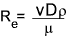For normal engineering calculations , the flow in pipes is considered laminar if the relevant Reynolds number is less than 2000, and it is turbulent if the Reynolds number is greater than 4000.  Between these two values there is the critical zone in which the flow can be either laminar or turbulent or the flow can change between the patterns...

It is important to know the type of flow in the pipe when assessing friction losses when determining the relevant friction factors

Reference :

The steady flow equation steady flow equation (energy per unit mass )  for a system is identified below...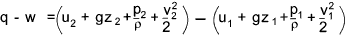If q = w = 0 and the fluid is incompressible and frictionless and if the variables are converted to measured heads of the fluid , that is the units are per unit weight (ρg) - then the Bernoulli's equation results ..
Reference .. Bernoulli's Equation ideal fluids
..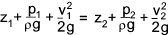In real flow systems there are losses due to internal and wall friction which result in increase in the internal energy of the fluid. (q > 0).   Reference Bernoulli's Equation real Fluids .    The bernoulli equation is modified to reflect these losses by adding a term h f = Head loss due to friction.= (u2 -u1 - q)   The modified bernoullis equation is therefore ..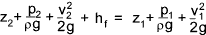The object of most pipe flow head loss calculations is to determine the friction head loss and allow estimation of the pump /compressor power required to pump the fluid along the piping.  In most fluid transfer cases the fluid is a incompressible (a liquid) and the flow rate (Q) is constant along the pipe run and therefore the velocity at any point can easily be calculated.  The head (z) can also be easily obtained from the pipeline geometry.  The system pressure and head loss are therefore the variables generally subject to the detailed pipeline calculations....

Pipe Flow Calculations

In determining the head loss (pressure drop) along a pipe as a result of friction losses it is first necessary to determine the following:
Diameter (m), Length (m), Fluid Viscosity( μ), Fluid density (ρ) and the fluid velocity (v). It is then necessary to obtain the relevant Reynolds number..

The equation for the Reynold NumberConsistent units to be used i.e Typically ρ = kg/m3, v = m/s, D= m, μ = Ns/m2 ( 1 Ns/m2 = 103cP)

The value for the Reynold number is to be used to evaluate if the flow is laminar or turbulent and can be used to obtain the friction factor " f " from a moody chart. The moody chart plots the friction factor (f) against the Reynold number with a number of different plotted lines for different values of absolute roughness/Diameter .

The head loss along the pipe can now be calculated using the Darcy-Weisbach equation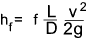The result of the calculation is in units of head of the fluid.  . It is based on the pipe being all one dia and the fluid is incompressible

For a single pipe line with a number of fittings the total head loss is calculated as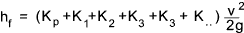K p = f (L/D) for the length of pipe. ( this may be made up of f(L/D). for a number of different pipe lengths of different diameters )
K 1..n = fT(L/D) equivalent for each fitting

A Moody chart (see below) is used to determine the turbulent flow friction factor from the Reynolds number and the relative roughness of the pipe. If the flow is laminar then the fricton factor is 64/Re.

Note: it is suggested that for laminar flow in pipe at Re number approaching 2000 the above K values are used for bends and fitting with reasonable accuracy

A moody chart and tables for roughness values and (L/D) factors for various fittings are provided below

Moody Chart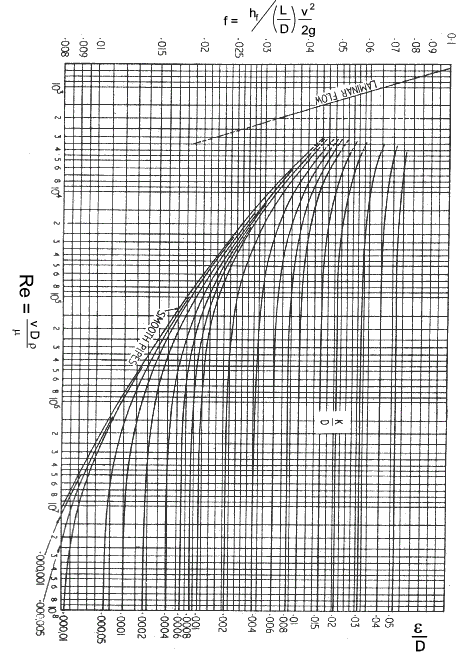Various typical values of hydraulic roughness (ε)

Note:
In the moody chart above (ε /D ) is identified with both numerator and denominator in metres (for consistency with all other equations on this page.   It is probably more convenient to express both in (mm).i.e a 50mm cast iron pipe (ε mm; /Dmm ) would simply be (0,203 /50 ).

 Type of Pipe ε .103..( = εmm ) Cast Iron 0,203 Galvanised Steel 0,152 Steel/Wrought Iron 0,051 Rivetted Steel 0,91 - 9,1 Asphalted Cast Iron 0,12 Wood-Stave 0,18 - 0,91 Concrete 3,0 Spun Concrete 0,203 Drawn Copper, Brass Steel,Glass Smooth

Typical Values of L/D for Fittings

The losses through fittings are generally evaluated by obtaining K = fT(L/D)

Table of pipe friction values for clean pipe in region of complete turbulence

 Nominal size(mm) 15 20 25 32 40 50 65,80 100 125 150 200,250 300,400 450,600 fT 0,027 0,025 0,023 0,022 0,021 0,019 0,018 0,017 0,016 0,015 0,014 0,013 0,012

 Fitting L/D Globe Valve 340 Gate Valve 8 Lift Check Valve 600 Swing Check Valve 50 - 100 Ball Valve 6 Butterfly Valve 35 Flush Pipe Entrance Sharp Corner K = 0.5 Flush Pipe Entrance radius >0,15 K = 0.04 Pipe Exit K = 1 Tee Through 20 Tee- Branch flow 60 Elbow-90 30 Elbow -45 16
 Fitting L/D Close Pattern Ret. Bend 50 90o Bend r /D=1 20 90o Bend r /D=2 12 90o Bend r /D=3 12 90o Bend r / D=6 17 90o Bend r / D=8 24 90o Bend r / D=10 30 90o Bend r / D=12 34 90o Bend r / D=14 38 90o Bend r / D=16 42 90o Bend r / D=16 46 90o Bend r / D=20 50

The K180 value for a 180o bend may be derived from the equivalent K90 which is calculated from the above tables using the equation

The K180 = 0,25.π.fT . r/D + 1,5.K90

For laminar fluids with low Re numbers ( "<" 500) the K values obtained using the above are probably very innaccurate. The table below illustrates how this affects the K values

 Fitting K values for low Reynolds Number fluids Re = 1000 500 100 50 90 deg Elbow Short Radius 0,9 1 7,5 16 Gate Valve 1,2 1,7 9,9 24 Globe Valve 11 12 20 30 Plug Valve 12 14 19 27 Angle Valve 8 8.5 11 19 Swing check Valve 4 4,5 17 55

K values for Sudden Expansion-Contraction & Orifice

The losses through these fitting are generally evaluated by first obtaining β = d2 / d1

Important Note: the resulting K values as tabled below are based on the flow velocity in the larger pipe if the flow velocity in the small pipe is used to evaluate the head loss then the K values tabled below should be multiplied by ( β ) 4 = (d2 / d1) 4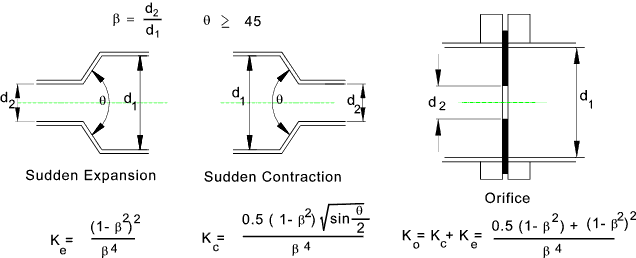Table of Ke,Kc & KO against   β = d2 / d1

 β K e K c K o β K e K c K o 0.15 1887.42 965.43 2852.85 0.6 3.16 2.47 5.63 0.2 576 300 876 0.65 1.87 1.62 3.49 0.25 225 120 345 0.7 1.08 1.06 2.14 0.3 102.23 56.17 158.4 0.75 0.6 0.69 1.29 0.35 51.31 29.24 80.55 0.8 0.32 0.44 0.76 0.4 27.56 16.41 43.97 0.85 0.15 0.27 0.42 0.45 15.51 9.72 25.23 0.9 0.06 0.14 0.2 0.5 9 6 15 0.95 0.01 0.06 0.07 0.55 5.32 3.81 9.13 1 0 0 0

Reasonable Velocities of fluid in Pipes

 Medium Pressure (bar) Service Velocity (m/s) Notes Steam (sat) 0 - 1.7 Heating 20 to 30 + 100mm dia Steam (sat) over 1.7 Process 30 to 50 +150mm dia Steam (sup) over 14 Process 30 to 100 +150mm dia Air Forced Air Flow 5 to 8 e.g. AC Reheat Water - General 1 to 3 Water Concrete Pipe 4,7 Water Pump Suction 1,2 Water Horizontal Sewer 0,75 Minimum Water Pump discharge 1,2 to 2,5 Minimum Water Boiler Feed 2,4 to 4,6 Minimum Oil Hydraulic Systems 2,1 to 4,6 Minimum Ammonia Compressor Suction 25 Max. Permissable Ammonia Compressor Discharge 30 Max. Permissible

 Useful Links Efluids .. Site includes lots of really useful information and calulculators Wikipedia Mechanics of Fluids .. Excellent source of information Efunda Pipe Pressure Loss Calculator .. Authoritative Calculator and notes 1MNO eng.. A site containing a large number of Fluid Flow Calculations and Calculators :Some of the calculators require registration for a fee; Engineering Page.. A site including various Engineering Calculators - Very good pump calculator Spirax Sarco...Excellent Reference Site . Learning centre includes fluid flow reference information

Fluids Index